## List of variables and their meaning

no *ELECTROMAGNETICS calculation *ELECTROMAGNETICS calculation variable meaning FILE NAMES (132 characters long) jobnamec(1) jobname jobnamec(2) INPUT file on *VIEWFACTOR card jobnamec(3) OUTPUT file on *VIEWFACTOR card jobnamec(4) INPUT file on *SUBMODEL card (global model) or on *CRACK PROPAGATION card jobnamec(5) FILENAME on *SUBSTRUCTURE MATRIX OUTPUT card (storage file for stiffness matrix) jobnamec(6) FILE on *TEMPERATURE card REARRANGEMENT OF THE ORDER IN THE INPUT DECK ifreeinp next blank line in field inp ipoinp(1,i) index of the first column in field inp containing information on a block of lines in the input deck corresponding to fundamental key i; a fundamental key is a key for which the order in the input file matters (the fundamental keys are listed in file keystart.f) ipoinp(2,i) index of the last column in field inp containing information on a block of lines in the input deck corresponding to fundamental key i; inp a column i in field inp (i.e. inp(1..3,i)) corresponds to a uninterrupted block of lines assigned to one and the same fundamental key in the input deck. inp(1,i) is its first line in the input deck, inp(2,i) its last line and inp(3,i) the next column in inp corresponding to the same fundamental key; it takes the value 0 if none other exists. MATERIAL DESCRIPTION nmat # materials matname(i) name of material i nelcon(1,i) # (hyper)elastic constants for material i (negative kode for nonlinear elastic constants) nelcon(2,i) # temperature data points for the elastic constants of material i elcon(0,j,i) temperature at (hyper)elastic temperature point j of material i elcon(k,j,i) (hyper)elastic constant k at elastic temperature point j of material i nelcon(1,i) # magnetic permeability constants for material i (always two) nelcon(2,i) # temperature data points for the magnetic permeability constants of material i elcon(0,j,i) temperature at magnetic permeability temperature point j of material i elcon(1,j,i) magnetic permeability at magnetic permeability temperature point j of material i elcon(2,j,i) domain of material i nrhcon(i) # temperature data points for the density of material i rhcon(0,j,i) temperature at density temperature point j of material i rhcon(1,j,i) density at the density temperature point j of material i nshcon(i) # temperature data points for the specific heat of material i shcon(0,j,i) temperature at temperature point j of material i shcon(1,j,i) specific heat at constant pressure at the temperature point j of material i shcon(2,j,i) dynamic viscosity at the temperature point j of material i shcon(3,1,i) specific gas constant of material i nalcon(1,i) # of expansion constants for material i nalcon(2,i) # of temperature data points for the expansion coefficients of material i alcon(0,j,i) temperature at expansion temperature point j of material i alcon(k,j,i) expansion coefficient k at expansion temperature point j of material i nalcon(1,i) # of electrical conductivity constants for material i (always 1) nalcon(2,i) # of temperature data points for the electrical conductivity constants of material i alcon(0,j,i) temperature at electrical conductivity temperature point j of material i alcon(1,j,i) electrical conductivity coefficient at electrical conductivity temperature point j of material i ncocon(1,i) # of conductivity constants for material i ncocon(2,i) # of temperature data points for the conductivity coefficients of material i cocon(0,j,i) temperature at conductivity temperature point j of material i cocon(k,j,i) conductivity coefficient k at conductivity temperature point j of material i orname(i) name of orientation i orab(1..6,i) coordinates of points a and b defining the new orientation orab(7,i) -1: cylindrical local system 1: rectangular local system norien # orientations nplicon(0,i) # temperature data points for the isotropic hardening curve of material i nplicon(j,i) # of stress - plastic strain data points at temperature j for material i plicon(0,j,i) temperature data point j of material i plicon(2*k-1,j,i) stress corresponding to stress-plastic strain data point k at temperature data point j of material i plicon(2*k-1,j,i) for springs: force corresponding to force-displacement data point k at temperature data point j of material i plicon(2*k-1,j,i) for penalty contact: pressure corresponding to pressure-overclosure data point k at temperature data point j of material i plicon(2*k,j,i) plastic strain corresponding to stress-plastic strain data point k at temperature data point j of material i for springs: displacement corresponding to force-displacement data point k at temperature data point j of material i for penalty contact: overclosure corresponding to pressure-overclosure data point k at temperature data point j of material i nplkcon(0,i) # temperature data points for the kinematic hardening curve of material i nplkcon(j,i) # of stress - plastic strain data points at temperature j for material i plkcon(0,j,i) temperature data point j of material i plkcon(2*k-1,j,i) stress corresponding to stress-plastic strain data point k at temperature data point j of material i for penalty contact: conductance corresponding to conductance-pressure data point k at temperature data point j of material i plkcon(2*k,j,i) plastic strain corresponding to stress-plastic strain data point k at temperature data point j of material i for penalty contact: pressure corresponding to conductance-pressure data point k at temperature data point j of material i kode=-1 Arrudy-Boyce -2 Mooney-Rivlin -3 Neo-Hooke -4 Ogden (N=1) -5 Ogden (N=2) -6 Ogden (N=3) -7 Polynomial (N=1) -8 Polynomial (N=2) -9 Polynomial (N=3) -10 Reduced Polynomial (N=1) -11 Reduced Polynomial (N=2) -12 Reduced Polynomial (N=3) -13 Van der Waals (not implemented yet) -14 Yeoh -15 Hyperfoam (N=1) -16 Hyperfoam (N=2) -17 Hyperfoam (N=3) -50 deformation plasticity -51 incremental plasticity (no viscosity) -52 viscoplasticity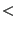-100 user material routine with -kode-100 user defined constants with keyword *USER MATERIAL PROCEDURE DESCRIPTION iperturb(1) = -1 : linear iteration in a nonlinear calculation = 0 : linear = 1 : second order theory for frequency/buckling/Green calculations following a static step (PERTURBATION selected)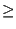2 : Newton-Raphson iterative procedure is active = 3 : nonlinear material (linear or nonlinear geometric and/or heat transfer) iperturb(2) 0 : linear geometric (NLGEOM not selected) 1 : nonlinear geometric (NLGEOM selected) nmethod 1 : static (linear or nonlinear) 2 : frequency(linear) 3 : buckling (linear) 4 : dynamic (linear or nonlinear) 5 : steady state dynamics 6 : Coriolis frequency calculation 7 : flutter frequency calculation 8 : magnetostatics 9 : magnetodynamics (inductive heating) 10 : electromagnetic eigenvalue problems 11 : superelement creation 12 : sensitivity analysis irstrt(1) 0: no restart calculation -1: RESTART,READ active; overwritten by write frequency while reading the restart file n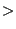0: write frequency; either read from a restart file with RESTART,READ or specified explicitly on a RESTART,WRITE card. irstrt(2) 0: no OVERLAY while writing a restart file 1: OVERLAY while writing a restart file iout governs the output and the calculation of the solution in results.c -2: v is assumed to be known and is used to calculate strains, stresses..., no result output; corresponds to iout=-1 with in addition the calculation of the internal energy density -1: v is assumed to be known and is used to calculate strains, stresses..., no result output; is used to take changes in SPC's and MPC's at the start of a new increment or iteration into account 0: v is calculated from the system solution and strains, stresses.. are calculated, no result output 1: v is calculated from the system solution and strains,stresses.. are calculated, requested results output 2: v is assumed to be known and is used to calculate strains, stresses..., requested results output GEOMETRY DESCRIPTION nk highest node number co(i,j) coordinate i of node j inotr(1,j) transformation number applicable in node j inotr(2,j) a SPC in a node j in which a transformation applies corresponds to a MPC. inotr(2,j) contains the number of a new node generated for the inhomogeneous part of the MPC TOPOLOGY DESCRIPTION ne highest element number mi(1) max # of integration points per element (max over all elements) mi(2) max degree of freedom per node (max over all nodes) in fields like v(0:mi(2))... if 0: only temperature DOF if 3: temperature + displacements if 4: temperature + displacements/velocities + pressure kon(i) field containing the connectivity lists of the elements in successive order for 1d and 2d elements (no composites) the 3d-expansion is stored first, followed by the topology of the original 1d or 2d element, for a shell composite this is followed by the topology of the expansion of each layer ipkon(i) (location in kon of the first node in the element connectivity list of element i)-1; for expanded elements the expanded connectivity comes first followed by the original 1d/2d connectivity lakon(i) element label C3D4: linear tetrahedral element (F3D4 for 3D-fluids) C3D6: linear wedge element (F3D6 for 3D-fluids) C3D6 E: expanded plane strain 3-node element = CPE3 C3D6 S: expanded plane stress 3-node element = CPS3 C3D6 A: expanded axisymmetric 3-node element = CAX3 C3D6 L: expanded 3-node shell element = S3 C3D8: linear hexahedral element (F3D8 for 3D-fluids) C3D8I: linear hexahedral element with incompatible modes C3D8 E: expanded plane strain 4-node element = CPE4 C3D8 S: expanded plane stress 4-node element = CPS4 C3D8 A: expanded axisymmetric 4-node element = CAX4 C3D8I L: expanded 4-node shell element = S4 C3D8I B: expanded 2-node beam element = B31 C3D8R: linear hexahedral element with reduced integration C3D8R E: expanded plane strain 4-node element with reduced integration = CPE4R C3D8R S: expanded plane stress 4-node element with reduced integration = CPS4R C3D8R A: expanded axisymmetric 4-node element with reduced integration = CAX4R C3D8R L: expanded 4-node shell element with reduced integration = S4R C3D8R B: expanded 2-node beam element with reduced integration = B31R C3D10: quadratic tetrahedral element C3D15: quadratic wedge element C3D15 E: expanded plane strain 6-node element = CPE6 C3D15 S: expanded plane stress 6-node element = CPS6 C3D15 A: expanded axisymmetric 6-node element = CAX6 C3D15 L: expanded 6-node shell element = S6 C3D15 LC: expanded composite 6-node shell element = S6 C3D20: quadratic hexahedral element C3D20 E: expanded plane strain 8-node element = CPE8 C3D20 S: expanded plane stress 8-node element = CPS8 C3D20 A: expanded axisymmetric 8-node element = CAX8 C3D20 L: expanded 8-node shell element = S8 C3D20 B: expanded 3-node beam element = B32 C3D20R: quadratic hexahedral element with reduced integration C3D20RE: expanded plane strain 8-node element with reduced integration = CPE8R C3D20RS: expanded plane stress 8-node element with reduced integration = CPS8R C3D20RA: expanded axisymmetric 8-node element with reduced integration = CAX8R C3D20RL: expanded 8-node shell element with reduced integration = S8R C3D20RLC: expanded composite 8-node shell element with reduced integration = S8R C3D20RB: expanded 3-node beam element with reduced integration = B32R GAPUNI: 2-node gap element ESPRNGA1 : 2-node spring element EDSHPTA1 : 2-node dashpot element ESPRNGC3 : 4-node contact spring element ESPRNGC4 : 5-node contact spring element ESPRNGC6 : 7-node contact spring element ESPRNGC8 : 9-node contact spring element ESPRNGC9 : 10-node contact spring element ESPRNGF3 : 4-node advection spring element ESPRNGF4 : 5-node advection spring element ESPRNGF6 : 7-node advection spring element ESPRNGF8 : 9-node advection spring element network elements (D-type):] DATR : absolute to relative DCARBS : carbon seal DCARBSGE : carbon seal GE (proprietary) DCHAR : characteristic DGAPFA : gas pipe Fanno adiabatic DGAPFAA : gas pipe Fanno adiabatic Albers (proprietary) DGAPFAF : gas pipe Fanno adiabatic Friedel (proprietary) DGAPFI : gas pipe Fanno isothermal DGAPFIA : gas pipe Fanno isothermal Albers (proprietary) DGAPFIF : gas pipe Fanno isothermal Friedel (proprietary) DLABD : labyrinth dummy (proprietary) DLABFSN : labyrinth flexible single DLABFSP : labyrinth flexible stepped DLABFSR : labyrinth flexible straight DLABSN : labyrinth single DLABSP : labyrinth stepped DLABSR : labyrinth straight DLDOP : oil pump (proprietary) DLICH : channel straight DLICHCO : channel contraction DLICHDO : channel discontinuous opening DLICHDR : channel drop DLICHDS : channel discontinuous slope DLICHEL : channel enlargement DLICHRE : channel reservoir DLICHSG : channel sluice gate DLICHSO : channel sluice opening DLICHST : channel step DLICHWE : channel weir crest DLICHWO : channel weir slope DLIPIBE : (liquid) pipe bend DLIPIBR : (liquid) pipe branch (not available yet) DLIPICO : (liquid) pipe contraction DLIPIDI : (liquid) pipe diaphragm DLIPIEL : (liquid) pipe enlargement DLIPIEN : (liquid) pipe entrance DLIPIGV : (liquid) pipe gate valve DLIPIMA : (liquid) pipe Manning DLIPIMAF : (liquid) pipe Manning flexible DLIPIWC : (liquid) pipe White-Colebrook DLIPIWCF : (liquid) pipe White-Colebrook flexible DLIPU : liquid pump DLPBEIDC : (liquid) restrictor bend Idelchik circular DLPBEIDR : (liquid) restrictor bend Idelchik rectangular DLPBEMA : (liquid) restrictor own (proprietary) DLPBEMI : (liquid) restrictor bend Miller DLPBRJG : (liquid) (liquid) branch joint GE DLPBRJI1 : (liquid) branch joint Idelchik1 DLPBRJI2 : (liquid) (liquid) branch joint Idelchik2 DLPBRSG : (liquid) (liquid) branch split GE DLPBRSI1 : (liquid) branch split Idelchik1 DLPBRSI2 : (liquid) branch split Idelchik2 DLPC1 : (liquid) orifice Cd=1 DLPCO : (liquid) restrictor contraction DLPEL : (liquid) restrictor enlargement DLPEN : (liquid) restrictor entry DLPEX : (liquid) restrictor exit DLPLOID : (liquid) restrictor long orifice Idelchik DLPLOLI : (liquid) restrictor long orifice Lichtarowicz DLPUS : (liquid) restrictor user DLPVF : (liquid) vortex free DLPVS : (liquid) vortex forced DLPWAOR : (liquid) restrictor wall orifice DMRGF : Moehring centrifugal DMRGP : Moehring centripetal DORBG : orifice Bragg (proprietary) DORBT : bleed tapping DORC1 : orifice Cd=1 DORMA : orifice proprietary, rotational correction Albers (proprietary) DORMM : orifice McGreehan Schotsch, rotational correction McGreehan and Schotsch DORPA : orifice Parker and Kercher, rotational correction Albers (proprietary) DORPM : orifice Parker and Kercher, rotational correction McGreehan and Schotsch DORPN : preswirl nozzle DREBEIDC : restrictor bend Idelchik circular DREBEIDR : restrictor bend Idelchik rectangular DREBEMA : restrictor own (proprietary) DREBEMI : restrictor bend Miller DREBRJG : branch joint GE DREBRJI1 : branch joint Idelchik1 DREBRJI2 : branch joint Idelchik2 DREBRSG : branch split GE DREBRSI1 : branch split Idelchik1 DREBRSI2 : branch split Idelchik2 DRECO : restrictor contraction DREEL : restrictor enlargement DREEN : restrictor entrance DREEX : restrictor exit DRELOID : restrictor long orifice Idelchik DRELOLI : restrictor long orifice Lichtarowicz DREUS : restrictor user DREWAOR : restrictor wall orifice DRIMS : rim seal (proprietary) DRTA : relative to absolute DSPUMP : scavenge pump (proprietary) DVOFO : vortex forced DVOFR : vortex free Uxxxxabc : user element with type number xxxx, number of integration points a, maximum degree of freedom in any node b and number of nodes c; a,b and c are calculated internally based on the information on the *USER ELEMENT card; notice that a, b and c are the character equivalent, e.g. a=char(number of integration points) ielorien(j,i) orientation number of layer j ielmat(j,i) material number of layer j ielprop(i) pointer to the position in field prop after which the properties for element i start (prop(ielprop(i)+1),prop(ielprop(i)+2)...); for networks and general beam sections nuel number of different user element types iuel(1,i) type number of the user element iuel(2,i) number of itegration points iuel(3,i) max degree of freedom in any of the nodes iuel 4,i) number of nodes belonging to the element SETS AND SURFACES nset number of sets (including surfaces) ialset(i) member of a set or surface: this is a - node for a node set or nodal surface - element for an element set - number made up of 10*(element number)+facial number for an element face surface if ialset(i)=-1 it means that all nodes or elements (depending on the kind of set) in between ialset(i-2) and ialset(i-1) are also member of the set set(i) name of the set; this is the user defined name + N for node sets + E for element sets + S for nodal surfaces + T for element face surfaces istartset(i) pointer into ialset containing the first set member iendset(i) pointer into ialset containing the last set member TIE CONSTRAINTS ntie number of tie constraints tieset(1,i) name of the tie constraint; for contact constraints (which do not have a name) the adjust nodal set name is stored, if any, and a C is appended at the end (C is replaced by - for deactivated contact pairs) for multistage constraints a M is appended at the end for a contact tie a T is appended at the end for submodels (which do not have a name) a fictitious name SUBMODELi is used, where i is a three-digit consecutive number and a S is appended at the end for sensitivity sets a D is appended at the end (for design variables) tieset(2,i) dependent surface name + S for nodal surfaces (node-to-face contact) + T for facial surfaces (node-to-face or face-to-face contact) + M for facial surfaces (quad-quad Mortar contact) + P for facial surfaces (quad-lin Petrov Galerkin Mortar contact) + G for facial surfaces (quad-quad Petrov Galerkin Mortar contact) + O for facial surfaces (quad-lin Mortar contact) tieset(3,i) independent surface name + T tietol(1,i) tie tolerance; used for cyclic symmetry ties special meaning for contact pairs:0 for large sliding0 for small sliding if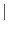tietol2, adjust value =tietol-2 tietol(2,i) for contact pairs: number of the relevant interaction definition (is treated as a material) for ties: -1 means ADJUST=NO, +1 means ADJUST=YES (default) tietol(3,i) only for contact pairs: the clearance defined in a *CLEARANCE card tietol(4,i) only for contact pairs:0 if reactivated with *MODEL CHANGE, ADD in the present step, else =0 CONTACT ncont total number of triangles in the triangulation of all independent surfaces ncone total number of slave nodes in the contact formulation mortar -2: no contact -1: massless dynamic contact 0: node-to-surface penalty contact 1: surface-to-surface penalty contact 2: mortar contact 3: linmortar contact 4: pglinmortar contact 5: pgmortar contact koncont(1..3,i) nodes belonging to the triangle koncont(4,i) element face to which the triangle belongs: 10*(element number) + face number cg(1..3,i) global coordinates of the center of gravity straight(1..4,i) coefficients of the equation of the plane perpendicular to the triangle and containing its first edge (going through the first and second node of koncont) straight(5..8,i) idem for the second edge straight(9..12,i) idem for the third edge straight(13..16,i) coefficients of the equation of the plane containing the triangle itietri(1,i) first triangle in field koncont of the master surface corresponding to contact tie constraint i itietri(2,i) last triangle in field koncont of the master surface corresponding to contact tie constraint i SHELL (2D) AND BEAM (1D) VARIABLES (INCLUDING PLANE STRAIN, PLANE STRESS AND AXISYMMETRIC ELEMENTS) iponor(2,i) two pointers for entry i of kon. The first pointer points to the location in xnor preceding the normals of entry i, the second points to the location in knor preceding the newly generated dependent nodes of entry i. The entry i relates to the unexpanded version of the element and, for element j, assumed to be stored at kon(ipkon(j)+1)...kon(ipkon(j)+m), where m is the number of 2d nodes belonging to the element xnor(i) field containing the normals in nodes on the elements they belong to knor(i) field containing the extra nodes needed to expand the shell and beam elements to volume elements thickn(2,i) thicknesses (one for shells, two for beams) in node i thicke(j,i) thicknesses (one (j=1) for non-composite shells, two (j=1,2) for beams and n (j=1..n) for composite shells consisting of n layers) in element nodes. The entries correspond to the nodal entries in field kon offset(2,i) offsets (one for shells, two for beams) in element i iponoel(i) pointer for node i into field inoel, which stores the 1D and 2D elements belonging to the node. inoel(3,i) field containing an element number, a local node number within this element and a pointer to another entry (or zero if there is no other). inoelfree next free field in inoel rig(i) integer field indicating whether node i is a rigid node (nonzero value) or not (zero value). In a rigid node or knot all expansion nodes except the ones not in the midface of plane stress, plane strain and axisymmetric elements are connected with a rigid body MPC. If node i is a rigid node rig(i) is the number of the rotational node of the knot; if the node belongs to axisymmetric, plane stress and plane strain elements only, no rotational node is linked to the knot and rig(i)=-1 AMPLITUDES nam # amplitude definitions amta(1,j) time of (time,amplitude) pair j amta(2,j) amplitude of (time,amplitude) pair j namtot total # of (time,amplitude) pairs amname(i) name of the amplitude namta(1,i) location of first (time,amplitude) pair in field amta namta(2,i) location of last (time,amplitude) pair in field amta namta(3,i) in absolute value the amplitude it refers to; if abs(namta(3,i))=i it refers to itself. If abs(namta(3,i))=j, amplitude i is a time delay of amplitude j the value of which is stored in amta(1,namta(1,i)); in the latter case amta(2,namta(1,i)) is without meaning; If namta(3,i)0 the time in amta for amplitude i is step time, else it is total time. TRANSFORMS ntrans # transform definitions trab(1..6,i) coordinates of two points defining the transform trab(7,i) =-1 for cylindrical transformations =1 for rectangular transformations SINGLE POINT CONSTRAINTS nboun # SPC's nodeboun(i) SPC node ndirboun(i) SPC direction typeboun(i) SPC type (SPCs can contain the nonhomogeneous part of MPCs) A=acceleration B=prescribed boundary condition M=midplane R=rigidbody U=usermpc xboun(i) magnitude of constraint at end of a step xbounold(i) magnitude of constraint at beginning of a step xbounact(i) magnitude of constraint at the end of the present increment xbounini(i) magnitude of constraint at the start of the present increment iamboun(i) amplitude number for submodels the step number is inserted ikboun(i) ordered array of the DOFs corresponding to the SPC's (DOF=8*(nodeboun(i)-1)+ndirboun(i)) ilboun(i) original SPC number for ikboun(i) MULTIPLE POINT CONSTRAINTS labmpc(i) label of MPC i j=ipompc(i) starting location in nodempc and coefmpc of MPC i nodempc(1,j) node of first term of MPC i nodempc(2,j) direction of first term of MPC i k=nodempc(3,j) next entry in field nodempc for MPC i (if zero: no more terms in MPC) coefmpc(j) first coefficient belonging to MPC i nodempc(1,k) node of second term of MPC i nodempc(2,k) direction of second term of MPC i coefmpc(k) coefficient of second term of MPC i ikmpc (i) ordered array of the dependent DOFs corresponding to the MPC's DOF=8*(nodempc(1,ipompc(i))-1)+nodempc(2,ipompc(i)) ilmpc (i) original MPC number for ikmpc(i) memmpc_ upper value of sum of number of terms in all MPC's mpcend last occupied entry in nodempc and coefmpc maxlenmpc maximum number of terms in any MPC icascade 0 : MPC's did not change since the last iteration 1 : MPC's changed since last iteration : dependency check in cascade.c necessary 2 : at least one nonlinear MPC had DOFs in common with a linear MPC or another nonlinear MPC. dependency check is necessary in each iteration FORCE CONSTRAINTS nfc number of force constraints coeffc(0,i) dof in the coupling surface corresponding to constraint i in the form dof=10*node+dir coeffc(1..6,i) coefficients for constraint i ndc number of distributing couplings ikdc(i) dof of distributing coupling i in the form dof=8*(refnode-1)+refdir idc(1,i) first constraint in field coeffc for distributing coupling i idc(2,i) last constraint in field coeffc for distributing coupling i idc(3,i) orientation for distributing coupling i POINT LOADS nforc # of point loads nodeforc(1,i) node in which force is applied nodeforc(2,i) sector number, if force is real; sector number + # sectors if force is imaginary (only for modal dynamics and steady state dynamics analyses with cyclic symmetry) ndirforc(i) direction of force xforc(i) magnitude of force at end of a step xforcold(i) magnitude of force at start of a step xforcact(i) actual magnitude iamforc(i) amplitude number idefforc(i) 0: no force was defined for this node and direction on the same sector before within the actual step 1: at least one force was defined for this node and direction on the same sector before within the actual step ikforc(i) ordered array of the DOFs corresponding to the point loads (DOF=8*(nodeboun(i)-1)+ndirboun(i)) ilforc(i) original SPC number for ikforc(i) FACIAL DISTRIBUTED LOADS nload # of facial distributed loads nelemload(1,i) element to which distributed load is applied nelemload(2,i) node for the environment temperature (only for heat transfer analyses); sector number, if load is real; sector number + # sectors if load is imaginary (only for modal dynamics and steady state dynamics analyses with cyclic symmetry) sideload(i) load label; indicated element side to which load is applied xload(1,i) magnitude of load at end of a step or, for heat transfer analyses, the convection (*FILM) or the radiation coefficient (*RADIATE) xload(2,i) the environment temperature (only for heat transfer analyses xloadold(1..2,i) magnitude of load at start of a step xloadact(1..2,i) actual magnitude of load iamload(1,i) amplitude number for xload(1,i) for submodels the step number is inserted iamload(2,i) amplitude number for xload(2,i) idefload(i) 0: no load was defined on the same element with the same label and on the same sector before within the actual step 1: at least one load was defined on the same element with the same label and on the same sector before within the actual step MASS FLOW RATE nflow # of network elements TEMPERATURE LOADS t0(i) initial temperature in node i at the start of the calculation t1(i) temperature at the end of a step in node i t1old(i) temperature at the start of a step in node i t1act(i) actual temperature in node i iamt1(i) amplitude number MECHANICAL BODY LOADS nbody # of mechanical body loads ibody(1,i) code identifying the kind of body load 1: centrifugal loading 2: gravity loading with known gravity vector 3: generalized gravity loading 4: Coriolis (for steady state dynamics) ibody(2,i) amplitude number for load i ibody(3,i) load case number for load i cbody(i) element number or element set to which load i applies xbody(1,i) size of the body load xbody(2..4,i) for centrifugal loading: point on the axis for gravity loading with known gravity vector: normalized gravity vector xbody(5..7,i) for centrifugal loading: normalized vector on the rotation axis xbodyact(1,i) actual magnitude of load xbodyact(2..7,i) identical to the corresponding entries in xbody idefbody(i) 0: no body load was defined on the same set with the same code and the same load case number before within the actual step 1: at least one body load was defined on the same set with the same code and the same load case number before within the actual step ipobody(1,i) body load applied to element i, if any, else 0 ipobody(2,i) index referring to the line in field ipobody containing the next body load applied to element i, i.e. ipobody(1,ipobody(2,i)), else 0 STRESS, STRAIN AND ENERGY FIELDS eei(i,j,k) in general : Lagrange strain component i in integration point j of element k (linear strain in linear elastic calculations) for elements with ⋆#star;DEFORMATION PLASTICITY property: Eulerian strain component i in integration point j of element k (linear strain in linear elastic calculations) eeiini(i,j,k) Lagrange strain component i in integration point of element k at the start of an increment een(i,j) Lagrange strain component i in node j (mean over all adjacent elements linear strain in linear elastic calculations) stx(i,j,k) Cauchy or PK2 stress component i in integration point j of element k at the end of an iteration (linear stress in linear elastic calculations). For spring elements stx(1..3,1,k) contains the relative displacements for element k and stx(4..6,1,k) the contact stresses sti(i,j,k) PK2 stress component i in integration point j of element k at the start of an iteration (linear stress in linear elastic calculations) stiini(i,j,k) PK2 stress component i in integration point j of element k at the start of an increment stn(i,j) Cauchy stress component i in node j (mean over all adjacent elements; "linear" stress in linear elastic calculations) ener(j,k) strain energy in integration point j of element k; ener(j,ne+k) kinetic energy in integration point j of element k; if k is a contact spring element: friction energy (j=1) enerini(j,k) strain energy in integration point of element k at the start of an increment enern(j) strain energy in node j (mean over all adjacent elements THERMAL ANALYSIS ithermal(1) 0 : no temperatures involved in the calculation (in this manual also 1 : stress analysis with given temperature field called ithermal) 2 : thermal analysis (no displacements) 3 : coupled thermal-mechanical analysis : temperatures and displacements are solved for simultaneously 4 : uncoupled thermal-mechanical analysis : in a new increment temperatures are solved first, followed by the displacements ithermal(2) used to determine boundary conditions for plane stress, plane strain and axisymmetric elements 0 : no temperatures involved in the calculation 1 : no heat transfer nor coupled steps in the input deck 2 : no mechanical nor coupled steps in the input deck 3 : at least one mechanical and one thermal step or at least one coupled step in the input deck v(0,j) temperature of node j at the end of an iteration (for ithermal1) vold(0,j) temperature of node j at the start of an iteration (for ithermal1) vini(0,j) temperature of node j at the start of an increment (for ithermal1) fn(0,j) actual temperature at node j (for ithermal1) qfx(i,j,k) heat flux component i in integration point j of element k at the end of an iteration qfn(i,j) heat flux component i in node j (mean over all adjacent elements) DISPLACEMENTS AND SPATIAL/TIME DERIVATIVES v(i,j) displacement of node j in direction i at the end of an iteration vold(i,j) displacement of node j in direction i at the start of an iteration vini(i,j) displacement of node j in direction i at the start of an increment ve(i,j) velocity of node j in direction i at the end of an iteration veold(i,j) velocity of node j in direction i at the start of an iteration veini(i,j) velocity of node j in direction i at the start of an increment accold(i,j) acceleration of node j in direction i at the start of an iteration accini(i,j) acceleration of node j in direction i at the start of an increment vkl(i,j) (i,j) component of the displacement gradient tensor at the end of an iteration xkl(i,j) (i,j) component of the deformation gradient tensor at the end of an iteration xikl(i,j) (i,j) component of the deformation gradient tensor at the start of an increment ckl(i,j) (i,j) component of the inverse of the deformation gradient tensor LINEAR EQUATION SYSTEM nasym 0: symmetrical system 1: asymmetrical system ad(i) element i on diagonal of stiffness matrix au(i) element i in lower triangle of stiffness matrix irow(i) row of element i in field au (i.e. au(i)) icol(i) number of subdiagonal nonzero's in column i (only for symmetric matrices) jq(i) location in field irow of the first subdiagonal nonzero in column i (only for symmetric matrices) adb(i) element i on diagonal of mass matrix, or, for buckling, of the incremental stiffness matrix (only nonzero elements are stored) aub(i) element i in upper triangle of mass matrix, or, for buckling, of the incremental stiffness matrix (only nonzero elements are stored) neq # of mechanical equations neq sum of mechanical and thermal equations neq neq + # of single point constraints (only for modal calculations) nzl number of the column such that all columns with a higher column number do not contain any (projected) nonzero off-diagonal terms (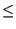neq) nzs sum of projected nonzero mechanical off-diagonal terms nzs nzs+sum of projected nonzero thermal off-diagonal terms nzs nzs + sum of nonzero coefficients of SPC degrees of freedom (only for modal calculations) nactdof(i,j)0: actual degree of freedom (in the system of equations) of DOF i of node j0 and even: -nactdof(i,j)/2 is the SPC number applied to this degree of freedom0 and odd: (-nactdof(i,j)+1)/2 is the MPC number for which this degree of freedom constitutes the dependent term inputformat =0: matrix is symmetric; lower triangular matrix is stored in fields ad (diagonal), au (subdiagonal elements), irow, icol and jq. =1: matrix is not symmetric. Diagonal and subdiagonal entries are stored as for inputformat=0; The superdiagonal entries are stored at the end of au in exactly the same order as the symmetric subdiagonal counterpart INTERNAL AND EXTERNAL FORCES fext(i) external mechanical forces in DOF i (due to point loads and distributed loads, including centrifugal and gravity loads, but excluding temperature loading and displacement loading) fextini(i) external mechanical forces in DOF i (due to point loads and distributed loads, including centrifugal and gravity loads, but excluding temperature loading and displacement loading) at the end of the last increment finc(i) external mechanical forces in DOF i augmented by contributions due to temperature loading and prescribed displacements; used in linear calculations only f(i) actual internal forces in DOF i due to : actual displacements in the independent nodes; prescribed displacements at the end of the increment in the dependent nodes; temperatures at the end of the increment in all nodes fini(i) internal forces in DOF i at the end of the last increment b(i) right hand side of the equation system : difference between fext and f in nonlinear calcultions; for linear calculations, b=finc. fn(i,j) actual force at node j in direction i INCREMENT PARAMETERS tinc user given increment size (can be modified by the program if the parameter DIRECT is not activated) tper user given step size dtheta normalized (by tper) increment size theta normalized (by tper) size of all previous increments (not including the present increment) reltime theta+dtheta dtime real time increment size time real time size of all previous increments INCLUDING the present increment ttime real time size of all previous steps DIRECT INTEGRATION DYNAMICS alpha parameter in the alpha-method of Hilber, Hughes and Taylor alpha if1: a transformation from a local rotating system to the global fixed system should be applied before starting the calculation else: no such transformation should be applied bet,gam parameters in the alpha-method of Hilber, Hughes and Taylor iexpl =0 : implicit dynamics =1 : explicit dynamics mscalmethod0: no explicit dynamics 0: explicit dyn., no mass nor contact spring scaling 1: explicit dyn., mass scaling, no contact spring scaling 2: explicit dyn., no mass scaling, contact spring scaling 3: explicit dyn., mass scaling and contact spring scaling smscale(i) scaling coefficient for element i if i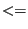ne0: mass scaling coefficient if ine0: contact spring scaling coefficient FREQUENCY CALCULATIONS mei number of requested eigenvalues mei number of Lanczos vectors mei maximum number of iterations mei if 1: store eigenfrequencies, eigenmodes, mass matrix and possibly stiffness matrix in .eig file, else 0 fei tolerance (accuracy) fei lower value of requested frequency range fei upper value of requested frequency range CYCLIC SYMMETRY CALCULATIONS mcs number of cyclic symmetry parts ics one-dimensional field; contains all independent nodes, one part after the other, and sorted within each part rcs one-dimensional field; contains the corresponding radial coordinates zcs one-dimensional field; contains the corresponding axial coordinates cs(1,i) number of segments in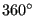cs(2,i) minimum nodal diameter cs(3,i) maximum nodal diameter cs(4,i) number of nodes on the independent side cs(5,i) number of sections to be plotted cs(6..12,i) coordinates of two points on the cyclic symmetry axis cs(13,i) number of the element set (for plotting purposes) cs(14,i) total number of independent nodes in all previously defined cyclic symmetry parts cs(15,i) cos(angle) where angle = 2*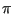/cs(1,mcs) cs(16,i) sin(angle) where angle = 2*/cs(1,mcs) cs(17,i) number of tie constraint MODAL DYNAMICS AND STEADY STATE DYNAMICS CALCULATIONS For Rayleigh damping (modal and steady state dynamics) xmodal(1)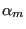(first Rayleigh coefficient) xmodal(2)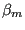(second Rayleigh coefficient) For steady state dynamics xmodal(3) lower frequency bound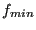xmodal(4) upper frequency bound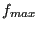xmodal(5) number of data points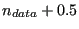xmodal(6) bias xmodal(7) if harmonic: -0.5; if not harmonic: number of Fourier coefficients + 0.5 xmodal(8) lower time bound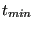for one period (nonharmonic loading) xmodal(9) upper time bound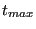for one period (nonharmonic loading) For damping (modal and steady state dynamics) xmodal(10) internal number of node set for which results are to be calculated xmodal(11) for Rayleigh damping: -0.5 for direct damping: largest mode for which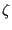is defined +0.5 For direct damping xmodal(12.. values of thecoefficients imddof(*) dofs which are retained (requested output, applied loads..) nmddof number of dofs in imddof imdnode(*) nodes which are retained (requested output, contact nodes..) nmdnode number of nodes in imdnode imdboun(*) boundary conditions needed at retained nodes nmdboun size of field imdboun imdmpc(*) MPCs needed at retained nodes nmdmpc size of field imdmpc imdelem(*) elements which are retained (calculation of stresses at the integration points....) nmdelem size of field imdelem iznode(*) nodes in imdnode + nodes with loading (user and non-user); only the results in the nodes in iznode are mapped onto the other sectors nznode size of field iznode izdof(*) retained dofs: dofs in imddof + dofs in nodes with non-user cloads and dloads; only those dofs are stored in field z nzdof size of field izdof OUTPUT IN .DAT FILE prset(i) node or element set corresponding to output request i prlab(i) label corresponding to output request i. It contains 6 characters. The first 4 are reserved for the field name, e.g. 'U ' for displacements, the fifth for the value of the TOTALS parameter ('T' for TOTALS=YES, 'O' for TOTALS=ONLY and ' ' else) and the sixth for the value of the GLOBAL parameter ('G' for GLOBAL=YES and 'L' for GLOBAL=NO). nprint number of print requests OUTPUT IN .FRD FILE filab(i) label corresponding to output field i. It contains 6 characters for the kind of output and 81 characters for the node set for which the output is requested, if any. The first 4 are reserved for the field name. The order is fixed: filab(1)='U ', filab(2)='NT ',filab(3)='S ',filab(4)='E ', filab(5)='RF ', filab(6)='PEEQ', filab(7)='ENER', filab(8)='SDV ', filab(9)='HFL ', filab(10)='RFL ', filab(11)='PU ', filab(12)='PNT ', filab(13)='ZZS ', filab(14)='TT ', filab(15)='MF ', filab(16)='PT ', filab(17)='TS ', filab(18)='PHS ', filab(19)='MAXU',filab(20)='MAXS', filab(21)='V ',filab(22)='PS ',filab(23)='MACH', filab(24)='CP ', filab(25)='TURB', filab(26)='CONT ' filab(27)='CELS ', filab(28)='DEPT ', filab(29)='HCRI ', filab(30)='MAXE', filab(31)='PRF ', filab(32)='ME ', filab(33)='HER, filab(34)='VF ', filab(35)='PSF ', filab(36)='TSF ', filab(37)='PTF ', filab(38)='TTF ', filab(39)='SF ', filab(40)='HFLF', filab(41)='SVF ', filab(42)='ECD ', filab(43)='POT ', filab(44)='EMFE', filab(45)='EMFB', filab(46)='PCON', filab(47)=SEN ', filab(48)='RM ' (the latter refers to mesh refinement), filab(49)='DEPF', filab(50)='DTF ', filab(51)='SNEG',filab(52)='SMID',filab(53)='SPOS', filab(54)='KEQ ',filab(55)='THE '. Results are stored for the complete mesh. A field is not selected if the first 4 characters are blank, e.g. the stress is not stored if filab(3)(1:4)=' '. An exception to this rule is formed for filab(1): here, only the first two characters are used and should be either 'U ' or ' ', depending on whether displacements are requested are not. The third character takes the value 'C' if the user wishes that the contact elements in each iteration of the last increment are stored in dedicated files, else it is blank. The fourth character takes the value 'I' if the user wishes that the displacements of the iterations of the last increment are stored (used for debugging in case of divergence), else it is blank. If the mesh contains 1D or 2D elements, the fifth character takes the value 'I' if the results are to be interpolated, 'M' if the section forces are requested instead of the stresses and 'E' if the 1D/2D element results are to be given on the expanded elements. In all other cases the fifth character is blank: ' '. The sixth character contains the value of the GLOBAL parameter ('G' for GLOBAL=YES and 'L' for GLOBAL=NO). The entries filab(13)='RFRES ' and filab(14)='RFLRES' are reserved for the output of the residual forces and heat fluxes in case of no convergence and cannot be selected by the user: the residual forces and heat fluxes are automatically stored if the calculation stops due to divergence. inum(i) =-1: network node =1: structural node or 3D fluid node CONVECTION NETWORKS ntg number of gas nodes itg(i) global node number nactdog(j,i) if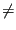0 indicates that degree of freedom j of gas node i is is an unknown; the nonzero number is the column number of the DOF in the convection system of equations. The physical significance of j depends on whether the node is a midside node or corner node of a fluid element: j=0 and corner node: total temperature j=1 and midside node: mass flow j=2 and corner node: total pressure j=3 and midside node: geometry (e.g.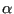for a gate valve) nacteq(j,i) if0 indicates that equation type j is active in gas node i; the nonzero number is the row number of the DOF in the convection system of equations. The equation type of j depends on whether the node is a midside node or corner node of a network element: j=0 and corner node: conservation of energy j=1 and corner node: conservation of mass j=2 and midside node: convervation of momentum ineighe(i) only for gas network nodes (no liquids): if 0: itg(i) is a midside node if -1: itg(i) is a chamber if0: ineighe(i) is a gas pipe element itg(i) belongs to v(j,i) value of degree of freedom j in node i (global numbering). The physical significance of j depends on whether the node is a midside node or corner node of a network element: j=0 and corner node: total temperature j=1 and midside node: mass flow j=2 and corner node: total pressure j=3 and corner node: static temperature j=3 and midside node: geometry nflow number of network elements ieg(i) global element number corresponding to network element i network if 0: no network if 1: defined as purely thermal by the user on the *STEP card (only unknowns: total temperature; simultaneous solution) if 2: purely thermal (alternating solution (presence of Dx-elements) or simultaneous (no Dx elements)) if 3: coupled thermodynamic network (alternating solution) if 4: purely aerodynamic (total temperature is known everywhere; alternating solution) THERMAL RADIATION ntr number of element faces loaded by radiation = radiation faces iviewfile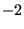: NO CHANGE option on the *VIEWFACTOR card, no reading, no calculating, no writing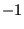: reading the viewfactors from file, no writing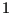: calculating the viewfactors, no writing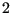: calculating the viewfactors, writing to file and continuing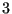: calculating the viewfactors, stop after storing the viewfactors to file kontri(1..3,j) nodes belonging to triangle j kontri(4,j) radiation face number (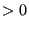andntri)to which triangle j belongs nloadtr(i) distributed load number (andnload) corresponding to radiation face i ITERATION VARIABLES istep step number iinc increment number iit iteration number = -1 only before the first iteration in the first increment of a step = 0 before the first iteration in an increment which was repeated due to non-convergence or any other but the first increment of a stepdenotes the actual iteration number PHYSICAL CONSTANTS physcon(1) Absolute zero physcon(2) Stefan-Boltzmann constant physcon(3) Newton Gravity constant physcon(4) Static temperature at infinity (for 3D fluids) physcon(5) Velocity at infinity (for 3D fluids) physcon(6) Static pressure at infinity (for 3D fluids) physcon(7) Density at infinity (for 3D fluids) physcon(8) Typical size of the computational domain (for 3D fluids) physcon(9) Turbulence parameter if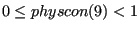: laminar if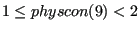: k-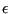Model if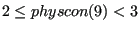: q-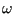Model if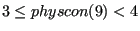: SST Model physcon(11) number of eigenvectors used in the creation of a random field physcon(12) standard deviation used in the creation of a random field physcon(13) correlation length used in the creation of a random field physcon(14) shock smoothing coefficient (CFD method) COMPUTATIONAL FLUID DYNAMICS vold(0,i) Static temperature in node i vold(1..3,i) Velocity components in node i vold(4,i) Pressure in node i voldaux(0,i) Total energy density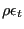in node i voldaux(1..3,i) Momentum density components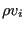in node i voldaux(4,i) Density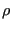in node i v(0,i) Total energy density correction in node i v(1..3,i) Momentum density correction components in node i v(4,i) For fluids: Pressure correction in node i For gas: Density correction in node i ELECTROMAGNETICS vold(0,i) Static temperature in node i vold(1..3,i) Magnetic vector potential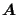(domain 2 or 3) in node i vold(4,i) Electric scalar potential V (domain 2) in node i vold(5,i) Magnetic scalar potential P (domain 1) in node i sti(1..3,j,k) Electric field in the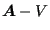domain (domain 2) in integration point j of element k sti(4..6,j,k) Magnetic field in any domain in integration point j of element k neq # of electromagnetic equations (covering the magnetic scalar potential in domain 1, the magnetic vector potential and electric scalar potential in domain 2 an the magnetic vector potential in domain 3) neq sum of electromagnetic and thermal equations nzs sum of projected nonzero electromagnetic off-diagonal terms nzs nzs+sum of projected nonzero thermal off-diagonal terms h0ref(1..3,i) magnetic intensity in infinite space due to the nominally applied electrical potential across the coils h0(1..3,i) magnetic intensity in infinite space due to the actually applied electrical potential across the coils CONVERGENCE PARAMETERS qa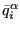for the mechanical forces (average force) qafor the thermal forces (average flux) qa -1: no time increment decrease0: time increment multiplication factor (1) due to divergence in a material routine qa maximum of the change of the viscoplastic strain within a given increment over all integration points in all elements qam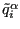for the mechanical forces qamfor the concentrated heat flux ram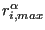for the mechanical forces ramfor the concentrated heat flux ram the node corresponding to ram ram the node corresponding to ram ram ram (present iteration) + ram (previous iteration ram number of contact elements (present iteration) - number of contact elements (previous iteration) uam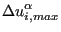for the displacements uamfor the temperatures cam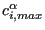for the displacements camfor the temperatures cam largest temperature change within the increment cam node corresponding to cam cam node corresponding to cam for networks uamt largest increment of gas temperature camt largest correction to gas temperature camt node corresponding to camt uamf largest increment of gas massflow camf largest correction to gas massflow camf node corresponding to camt uamp largest increment of gas pressure camp largest correction to gas pressure camp node corresponding to camt iflagact 0: number of contact elements did not significantly change between present and prevous iteration 1: else (i.e. did significantly change) THREE-DIMENSIONAL INTERPOLATION cotet(1..3,i) coordinates of nodes i kontet(1..4,i) nodes belonging to tetrahedron i ipofa(i) entry in field inodfa pointing to a face for which node i is the smallest number inodfa(1..3,i) nodes j, k and l belonging to face i such that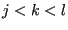inodfa(4,i) number of another face for which inodfa(1,i) is the smallest number. If no other exists the value is zero planfa(1..4,i) coefficients a, b, c and d of the plane equation ax+by+cz+d=0 of face i ifatet(1..4,i) faces belonging to tetrahedron i. The sign identifies the half space to which i belongs if evaluating the plane equation of the face

It is important to notice the difference between cam and cam. cam is the largest change within an iteration of the actual increment. If the corrections in subsequent iterations all belonging to the same increment are 5,1,0.1, the value of cam is 5. cam is the largest temperature change within the increment, in the above example this is 6.1.

 tieset(1) tieset(1) tieset(2) tieset(3) meaning (1:80) (81:81) Adjust node C Slave set Master set Contact set Tie name M Slave set Master set Multistage Tie name P Slave set Master set Fluid periodic Tie name Slave set Master set Cyclic symmetry Type D Design variable Design variables (COORDINATE or node set ORIENTATION) Tie name T Slave set Master set Tie S (1:10) Number of (1:10) Number Submodel node or face set of global (11:11) type ('N' for element set nodal, 'T' for face) Tie name Z Slave set Master set Fluid cyclic